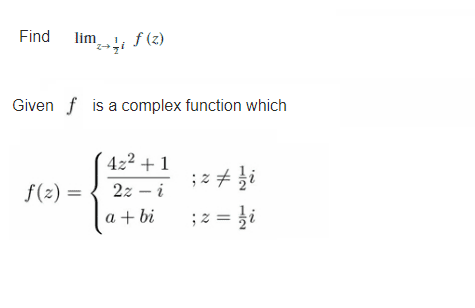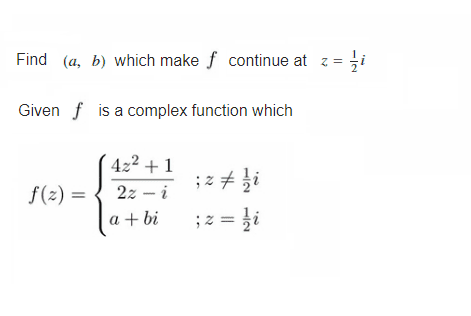# Complex number function

• MHB
• jaychay
You are correct. Substitute the hint DaalChawal gave into the function definition of $f(z)$ and simplify it.Then substitute the limit value $z=\frac 12 i$ for $f(z)$ with $z\ne \frac 12 i$ and evaluate it.How far do you get?

#### jaychayCan you help me with this two questions
I am really struggle on how to do it

Factorize $(4z^2 + 1) = (4z^2 - i^2)= (2z+i)(2z-i)$

DaalChawal said:
Factorize $(4z^2 + 1) = (4z^2 - i^2)= (2z+i)(2z-i)$
How can I solve the limit

jaychay said:
How can I solve the limit
Substitute the hint DaalChawal gave into the function definition of $f(z)$ and simplify it.
Then substitute the limit point $z=\frac 12 i$ for $f(z)$ with $z\ne \frac 12 i$ and evaluate it.
How far do you get?

Last edited:
Klaas van Aarsen said:
Substitute the hint DaalChawal gave into the function definition of $f(z)$ and simplify it.
Then substitute the limit value $z=\frac 12 i$ for $f(z)$ with $z\ne \frac 12 i$ and evaluate it.
How far do you get?
On question 2
Can you tell me on how to find (a,b) that can make it continue at z=1/2i

jaychay said:
On question 2
Can you tell me on how to find (a,b) that can make it continue at z=1/2i
Choose $a$ and $b$ such that $a+bi$ is the same as the limit value from question 1.
Did you find the limit value or are you stuck somewhere?

You made a mistake there bro
$2(\frac{i}{2})+i = 2i$

jaychay said:
Did I do it correct ?
The first substitution is correct, but the parentheses are placed the wrong way in the substitution of $z=\frac 12 i$.
It should be:
$$\lim_{z\to\frac 12i} f(z) = \lim_{z\to\frac 12i} \frac{4z^2+1}{2z-i} = \ldots = \lim_{z\to\frac 12i} 2z+i = 2\left(\frac 12 i\right) +i=2i$$

Tha
DaalChawal said:
You made a mistake there bro
$2(\frac{i}{2})+i = 2i$
Thank you bro

Klaas van Aarsen said:
Substitute the hint DaalChawal gave into the function definition of $f(z)$ and simplify it.
Then substitute the limit point $z=\frac 12 i$ for $f(z)$ with $z\ne \frac 12 i$ and evaluate it.
How far do you get?
Can you please check me on my another post on complex graph equation problem on question 2 on how to find ( a, b )

Klaas van Aarsen said:
The first substitution is correct, but the parentheses are placed the wrong way in the substitution of $z=\frac 12 i$.
It should be:
$$\lim_{z\to\frac 12i} f(z) = \lim_{z\to\frac 12i} \frac{4z^2+1}{2z-i} = \ldots = \lim_{z\to\frac 12i} 2z+i = 2\left(\frac 12 i\right) +i=2i$$
On question 2
Is ( a,b ) equal to ( 0,2 ) right ?

Klaas van Aarsen said:
The first substitution is correct, but the parentheses are placed the wrong way in the substitution of $z=\frac 12 i$.
It should be:
$$\lim_{z\to\frac 12i} f(z) = \lim_{z\to\frac 12i} \frac{4z^2+1}{2z-i} = \ldots = \lim_{z\to\frac 12i} 2z+i = 2\left(\frac 12 i\right) +i=2i$$
Klaas van Aarsen said:
Substitute the hint DaalChawal gave into the function definition of $f(z)$ and simplify it.
Then substitute the limit point $z=\frac 12 i$ for $f(z)$ with $z\ne \frac 12 i$ and evaluate it.
How far do you get?
On question 2
Is ( a,b ) equal to ( 0,2 ) right ?